Next: A Special Case Up: Proof that Algorithm Increases Previous: Corollary 1.2   Contents

### Theorem 2

Theorem 2: Let the state vector before step 2a of Grover's algorithm be as follows:

• For the unique marked state Sm which satisfies C(Sm) = 1 the amplitude is k such that 0 < k < 1/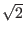• For each of the remaining (N - 1) states the amplitude is l such that l > 0
In this case we seek to prove both:
• The change in k,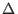k after steps 2a and 2b in Grover's algorithm is bounded below byk >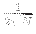• After steps 2a and 2b in Grover's algorithm l > 0

Proof: Let the initial amplitudes be k and l, let the amplitudes after the selected phase inversion step 2a be k' and l', let the amplitudes after the inversion about average step 2b be k'' and l''.

By theorem 1 we know k'' =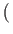1 -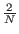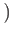k + 2l (note the reversal of terms in the coefficient of k, this is due to the phase inversion of k in step 2a), thereforek = k'' - k = -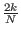+2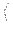1 -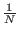l. By the assumption 0 < k < 1/and Corollary 1.2 it follows that | l| >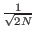. By assumption l is positive, thus l >. Combining this withk = k'' - k = -+21 -l. it follows thatk >. [Grover96]

To show l'' positive consider after step 2a of the algorithm, after the selective phase inversion, but before the inversion about average. At this point k' < 0 and l' > 0, since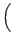0 < k <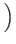and | l| >(from previous paragraph) that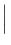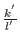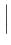<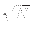. This means that after step 2a our register is in a state covered by Corollary 1.1, which states after the inversion about average operation l'' will be positive.Next: A Special Case Up: Proof that Algorithm Increases Previous: Corollary 1.2   Contents
Matthew Hayward - Quantum Computing and Grover's Algorithm GitHub Repository